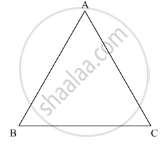Advertisement Remove all ads

# Is the Following Statement True and False : If One Angle of a Triangle is Obtuse, Then It Cannot Be a Right Angled Triangle. - Mathematics

MCQ
True or False

Is the following statement true and false :

If one angle of a triangle is obtuse, then it cannot be a right angled triangle.

#### Options

• Ture

• False

Advertisement Remove all ads

#### Solution

If one angle of a triangle is obtuse, then it cannot be a right angles triangle.According to the angle sum property of the triangle

In ΔABC

∠A +∠B + ∠C = 180°

Now, if it is a right angled triangle

Then,

∠A + ∠B + ∠C = 180°

90° + ∠B + ∠C =180°

∠B +∠C = 90°

Also if one of the angle’s is obtuse

∠B +∠C > 90°

This is not possible.

Thus, if one angle of a triangle is obtuse, then it cannot be a right angled triangle.

Is there an error in this question or solution?
Advertisement Remove all ads

#### APPEARS IN

RD Sharma Mathematics for Class 9
Chapter 11 Triangle and its Angles
Exercise 11.2 | Q 6.08 | Page 21
Advertisement Remove all ads
Advertisement Remove all ads
Share
Notifications

View all notifications

Forgot password?
Course